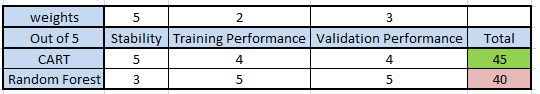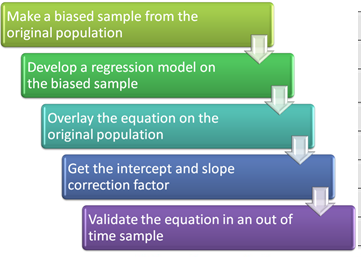## Decision Tree Algorithms Simplified 2

One of the advantage of using Decision tree is that it efficiently identifies the most significant variable and splits the population on it. In previous article, we developed a high level understanding of Decision trees. In this article, we will focus on the science behind splitting the nodes and choosing the most significant split.Decision trees can use various algorithms to split a node in two or more sub-nodes. The creation of sub-nodes increases the homogeneity of resultant sub-nodes. In## Decision Tree Simplified!

What is a Decision Tree? Decision tree is a type of supervised learning algorithm (having a pre-defined target variable) that is mostly used in classification problems. It works for both categorical and continuous input and output variables. In this technique, we split the population or sample into two or more homogeneous sets (or sub-populations) based on most significant splitter / differentiator in input variables.Example:-Let’s say we have a sample of 30 students with three variables Gender## Comparing a Random Forest to a CART model part 2

Random forest is one of the most commonly used algorithm in Kaggle competitions. Along with a good predictive power, Random forest model are pretty simple to build. We have previously explained the algorithm of a random forest ( Introduction to Random Forest ). This article is the second part of the series on comparison of a random forest with a CART model. In the first article, we took an example of an inbuilt R-dataset to predict the classification of an specie. In this article we will build a## Comparing a CART model to Random Forest (Part 1)

I created my first simple regression model with my father in 8th standard (year: 2002) on MS Excel. Obviously, my contribution in that model was minimal, but I really enjoyed the graphical representation of the data. We tried validating all the assumptions etc. for this model. By the end of the exercise, we had 5 sheets of the simple regression model on 700 data points. The entire exercise was complex enough to confuse any person with average IQ level. When I look at my models today, which areOnly 531 out of a population of 50,431 customer closed their saving account in a year, but the dollar value lost because of such closures was more than \$ 5 Million.The best way to arrest these attrition was by predicting the propensity of attrition for individual customer and then pitch retention offers to these identified customers. This was a typical case of modeling in a rare event population. This kind of problems are also very common in Health care analytics.In such analysis, there are two